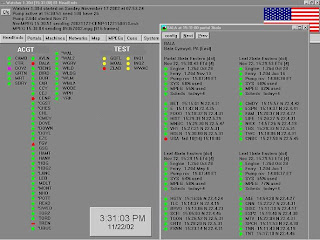## Thursday, September 11, 2008

### How the Flag got into WATCHER

What is WATCHER? If you have been reading my novel, you know that is the name of the marvellous program which the Control Room Guys of AC&TG use to keep watch over the activities and status of the Field - the 200-odd remote computer systems which do the ad insertion of local spots onto cable television. In the real world, it was the same thing. Of course it does not exist any more. For details on that, see here and here.

Anyway, I was able to find a snapshot of a WATCHER screen for you to see:It is interesting because of the American flag in the upper right. It was just seven years ago tomorrow that it was added to the software. I have written a short story about how that happened - it is the story of what really happened there, just slightly altered to let our hero Joe Outis be its main character, though anachronistic, since in the novel he only started work in 2004. (But that's how fiction works, hee hee.)

So if you are a literary person, you can enjoy that story. But if you are a tech person, you might wish to see what it was "Doc" was typing, and I decided to show you that as well. And it has the heraldic stuff too, which makes it even more fun than usual. Unfortunately, it does not come across very well, for the programming language called "C" requires careful use of indenting, like a fine poem. Not that "C" is poetic, except in the Greek sense! But perhaps some students of heraldry will appreciate it. Note: I am aware there are better ways of doing the stars. You have to remember I was writing this while I was talking with - uh - "Joe"... and on the day after 9/11.

Yes, for real. But obviously it was something worth doing, and WATCHER showed the flag for the first 10 seconds of every minute, as long as the systems continued to run, in fitting memory of September 11...

--Dr. Thursday

PS: note this is the real code, but there are other pieces of the gearwork one will need. So you're on your own if you wish to try to make it functional. I'd help, but you might not want to pay for it.

`////////////////////////////////////////////////////////////////////////////////////////// draw a star on the hdc centered at (xc, yc) with radius rvoid ShowStar(HDC hdc,int xc,int yc,int r){ int i; double a,aa,xx,yy; POINT poly; HRGN reg; int factor={0,2,4,1,3}; if(r<5) { poly.x=xc; poly.y=yc+r; poly.x=xc+r; poly.y=yc; poly.x=xc; poly.y=yc-r; poly.x=xc-r; poly.y=yc; reg=CreatePolygonRgn(poly,4,ALTERNATE); } else { for(i=0;i<5;i=i+1) { a=90.0+factor[i]*72; aa=a*pi/180.0; xx=r*cos(aa); yy=r*sin(aa); poly[i].x=xc+(int)xx; poly[i].y=yc-(int)yy; }//end for reg=CreatePolygonRgn(poly,5,WINDING); } FillRgn(hdc,reg,WhiteBrush); DeleteObject(reg);}////////////////////////////////////////////////////////////////////////////////////////// draw the flag of the United States on hdc within the given rectanglevoid ShowFlag(HDC hdc,int xlo,int ylo,int xhi,int yhi){ POINT poly; HRGN reg; int i,j,bar; double dx,dy,xm,ym,r,ddx,ddy,x,y; // barry of 13 gules and argent... xm=(xhi-xlo)/2; bar=(yhi-ylo)/13; ym=7*bar; for(i=0;i < 13;i=i+1) { if(i < 5) { poly.x=xlo+(long)xm; poly.y=ylo+i*bar; poly.x=xhi; poly.y=ylo+i*bar; poly.x=xhi; poly.y=ylo+i*bar+bar; poly.x=xlo+(long)xm; poly.y=ylo+i*bar+bar; } else { poly.x=xlo; poly.y=ylo+i*bar; poly.x=xhi; poly.y=ylo+i*bar; poly.x=xhi; poly.y=ylo+i*bar+bar; poly.x=xlo; poly.y=ylo+i*bar+bar; } reg=CreatePolygonRgn(poly,4,ALTERNATE); if(i&1) FillRgn(hdc,reg,WhiteBrush); else FillRgn(hdc,reg,RedBrush); DeleteObject(reg); }//end for // a canton azure... poly.x=xlo; poly.y=ylo; poly.x=xlo+(long)xm; poly.y=ylo; poly.x=xlo+(long)xm; poly.y=ylo+(long)ym; poly.x=xlo; poly.y=ylo+(long)ym; reg=CreatePolygonRgn(poly,4,ALTERNATE); FillRgn(hdc,reg,BlueBrush); DeleteObject(reg); // with 50 mullets argent... dx=xm/6; dy=ym/5; if(dx < dy) r=dx/3; else r=dy/3; r=3*r/4; for(i=0;i<6;i=i+1) { x=xlo+dx/2+dx*i; for(j=0;j<5;j=j+1) { y=ylo+dy/2+dy*j; ShowStar(hdc,(int)x,(int)y,(int)r); }//end for }//end for ddx=(xm-2*dx)/4; ddy=(ym-2*dy)/3; for(i=0;i<5;i=i+1) { x=xlo+dx+ddx*i; for(j=0;j<4;j=j+1) { y=ylo+dy+ddy*j; ShowStar(hdc,(int)x,(int)y,(int)r); }//end for }//end for}////////////////////////////////////////////////////////////////////////////////////////`